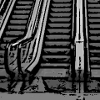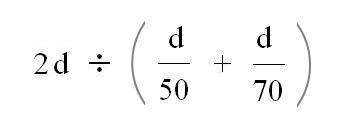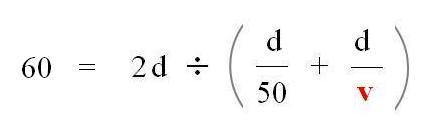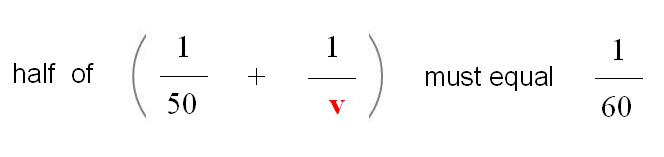#### You may also like### On the Road

Four vehicles travelled on a road. What can you deduce from the times that they met?### There and Back

Brian swims at twice the speed that a river is flowing, downstream from one moored boat to another and back again, taking 12 minutes altogether. How long would it have taken him in still water?### Escalator

At Holborn underground station there is a very long escalator. Two people are in a hurry and so climb the escalator as it is moving upwards, thus adding their speed to that of the moving steps. ... How many steps are there on the escalator?

# An Average Average Speed

##### Age 14 to 16Challenge Level

Well done Shaan from Watford Grammar School and many others who sent in well-explained correct solutions for this problem.

I noticed that neither the distance nor the journey time were given.

Time there was d / 50

Time back was d / 70

The Average speed for the round trip was total distance divided by total timeThe d cancels, indicating that the average speed does not depend on the distance so long as there and back are equal distances, and the answer is 58.3 (recurring) mph. which isn't sixty !
If the average speed is to be 60, I need to find v so that :which solves to give a v value of 75

And so 75 mph must be the return average speed if the overall average speed for the round trip is to be 60 mph.

Gemma in Suffolk added this thought :

The reason the average of the average speeds isn't the overall average speed is because the journey time isn't the same there and back . . . . but the distance is, and that's useful if instead I use the reciprocal of the rate.

Speed is a rate (the rate at which distance changes as time passes) and any rate can be considered in it's reciprocal form (the rate at which time passes per unit distance)

The distance is the same so adding the reciprocal of the speeds and then dividing by two does give me the reciprocal of the overall average speed.and this gives the answer 75 mph too.